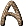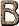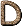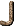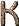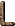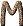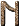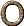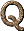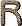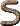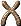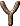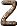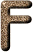F Spectral type for yellow-white stars, which are slightly hotter than the Sun. The brightest F-type stars in Earth's sky are Canopus and Procyon. [C95] F component The outer part of the Solar corona (see K Component) which emits a continuous spectrum in which absorption lines can be seen. The F corona is caused by radiation from the photosphere scattered by interplanetary dust, and it decreases slowly with distance from the Sun. (F stands for Fraunhofer) [H76] F Corona see F Component. [H76] FDS Law see Fermi-Dirac-Sommerfeld Law. [H76] f-Electron An orbital electron whose l quantum number is 3. [H76] F Layers Two layers in the Earth's ionosphere (F1 and F2 at about 200 and 300 km, respectively) immediately above the E layer. (also called Appleton Layers) [H76] f Number Ratio of the focal length to the diameter of a lens. [H76] F Region Region of the ionosphere above the F layers. [H76] F-Spot see Sunspots [H76] F Star A star of spectral type F with a surface temperature of about 60O0-7500 K, in which lines of hydrogen and Ca II are of about equal strength. Metal lines also become noticeable. Examples are Canopus, Procyon. [H76] f-Sum Rule The sum of the f-values for all the transitions from a given state (positive for absorption and negative for emission) is unity. [H76] f-Values see Oscillator Strengths [H76] ft-Values t = half-life of the-unstable nucleus, and f stands for an integral which depends on the-decay energy and the type of transition. [H76] Faber-Jackson Relation An empirically observed correlation between the speeds of stars in the center of a galaxy and the intrinsic luminosity of the galaxy - the higher the random speeds, the more luminous the galaxy. Since the speeds of stars can be directly measured by the Doppler shift in their colors, the Faber-Jackson relation permits an estimate of the intrinsic luminosity of a galaxy. By comparison of this with the observed brightness, the distance to the galaxy may be inferred. [LB90] Fabry-Perot Interferometer (a) A type of interferometer wherein the beam of light is passed through a series of pairs of partly reflecting surfaces set at various angles to it and spaced at certain prechosen numbers of the wavelength to be examined. It differs from the Michelson interferometer in that it has only one arm. [H76] (b) A device that measures distances and changes of distance very accurately, using the pattern of overlap of waves of light. [LB90] Faculae (a) Bright areas on the face of the Sun, commonly in the vicinity of sunspots. Named by Johannes Hevelius, they are thought to be caused by luminous hydrogen clouds close to the photosphere. They last on average about 15 Earth-days. [A84] (b) Bright regions of the photosphere seen in white light, visible only near the limb of the Sun. [H76] Faint Blue Galaxy A distant, irregularly shaped galaxy in which a large amount of star formation is taking place. [C97] Fall A "fall" as opposed to a "find" is a meteorite whose arrival on Earth is witnessed. Stones constitute 92% of the observed falls. [H76] False Color The use of colors, instead of shades of grey, on a computer image display screen to represent different brightness levels and highlight very small differences in a dramatic way. For example, in an ordinary black-and-white image, objects which differ only slightly in brightness appear as almost the same shade of grey and are hard to distinguish. If instead the numerical brightness values are assigned carefully chosen colors then two objects of almost equal brightness will be strongly distinguished when the image is displayed. [McL97] False Vacuum (a) A region of space that appears to be empty (a vacuum), but actually contains stored energy. When this stored energy is released, the false vacuum is said to decay. (see Vacuum) [LB90] (b) A peculiar state of matter which has never been observed, but which is predicted to exist by many modern theories of elementary particles, including the grand unified theories; like the ordinary vacuum, a false vacuum has no structure, so motion through a false vacuum cannot be detected; unlike the ordinary vacuum, a false vacuum has a large (positive) energy density and a large negative pressure; a false vacuum is the driving force behind the rapid expansion in the inflationary Universe model. (see Inflationary Universe) [D89] (c) The false vacuum is a peculiar form of matter which is predicted to exist by many (probably most) current theories of elementary particles. Particle physicists describe the false vacuum, like all forms of matter, in terms of fields. Originally the phrase "false vacuum" was used to describe a region in which one or more fields have a set of values that do not minimize the energy density, but which are in a local valley of the energy density diagram. Classically such a state would be absolutely stable, since there would be no energy available for the fields to jump over the hills that surround the valley. By the rules of quantum theory, however, the fields can tunnel through the hill of the energy density diagram and settle eventually in the true vacuum, the state of lowest energy density. The false vacuum is nonetheless metastable, and in fact it can endure for a period which is long by early Universe standards. The false vacuum has a negative pressure which creates a repulsive gravitational field, capable of driving the Universe into a period of exponential expansion called inflation. Similar effects occur if the fields are at the top of a broad plateau, instead of in a valley, so in some cases the phrase "false vacuum" includes this case as well. [G97] False Vacuum Bubble A bubble which has false vacuum on the inside, and true vacuum on the outside. In principle, the creation of such a bubble offers the possibility of creating a new Universe in a hypothetical laboratory. [G97] Families (a) Organization of matter particles into three groups, with each group being known as a family. The particles in each successive family differ from those in the previous by being heavier, but carry the same electric and nuclearforce charges. [G99] (b) Quarks and leptons appear to come in three families, although only one family appears to be needed to explain the world we see. The other families differ only in that they are heavier. We do not yet understand why there are three families, but we can fully describe their behavior. This is one of the main mysteries of particle physics. [K2000] Fanaroff-Riley ClassificationFano-Noise Fano-noise-limited CCDs used for X-ray detection are limited by intrinsic noise due to the absorption of energy and vibration of the crystal lattice itself. [McL97] Far Field The field of a pulsar beyond the velocity-of-light radius. [H76] Faraday Effect An effect occurring in HII regions in which a magnetic field causes a change in the polarized waves passing through (see Faraday Rotation). [H76] Faraday Rotation Rotation of the plane of polarization of linearly polarized radiation when the radiation passes through a plasma containing a magnetic field having a component in the direction of propagation. [H76] Feautrier's Method A difference-equation method of solving transfer equations. [H76] Fechner's Law The intensity of a sensation increases as the logarithm of the stimulus. (see Pogson's Ratio.) [H76] Feed Horn A device used on a radio telescope. It is located at the focal point and acts as a receiver of radio waves which the antenna collects and focuses on it. It couples the energy into the lines to go into the amplifier. [H76] Femto A prefix meaning 10-15. [H76] Fermi (a) A unit of length equal to 10-13 cm. [H76] (b) A unit of distance equal to 10-15 m. It is used for describing nuclear distances just as the ångström is used for atomic distances. It is named after E. Fermi (1901-1954), who built the first atomic pile; the unit was first used in 1956. [JM92] Fermi-Dirac Nuclei Nuclei of odd A-number (i.e., nuclei that do not have integral spin) (cf. Bose-Einstein nuclei). Fermi-Dirac nuclei therefore obey the Exclusion Principle. [H76] Fermi-Dirac-Sommerfeld Law A law which gives the algebraic number of a quantized system of particles which have velocities within a small range. [H76] Fermi Constant The parameter that fixes the strength with which the weak force couples to particles of matter in Fermi's original theory of the weak interaction. [D89] Fermi Gas A gas of electrons (or, more generally, fermions) in which all the lowest quantum states are occupied. For such a gas, the pressure in the nonrelativistic limit is proportional to the 5/3 power of the density. [H76] Fermi Interaction A weak interaction causing beta-decay. [H76] Fermilab The Fermi National Accelerator Laboratory, in Batavia, Illinois, USA. Fermilab is the home of the Tevatron, the world's most powerful accelerator, a pcollider with a maximum collision energy of 1.8 Te V (= 1800 Ge V = 1.8 × 1012 eV). [CD99] Fermi Level The maximum energy of any particle in a group of low-temperature subatomic particles called fermions. Fermions, such as electrons, cannot occupy the same space at the same energy. Thus, if many fermions are placed close together, their energies must all be different. The energy of that particle with the largest energy is the Fermi energy of the system. [LB90] Fermion (a) Any particle with half-integer spin: 1/2, 3/2, 5/2, etc. All fermions obey Pauli's exclusion principle. [CD99] (b) A particle that obeys Fermi-Dirac statistics and has half-integral spin. Elementary fermions are conserved in number in all interactions. The production of a new fermion is always accompanied by the production of an antiparticle which is counted as minus the particle. The lepton is one type of fermion which appears most often in the charged form, the electron. When an electron is created in beta decay an antineutrino is also produced. This can be regarded as an uncharged antiparticle, and is counted as minus one lepton. The positron is regarded as a charged antiparticle to the electron. When electron-positron pairs are generated by radiation or annihilate to radiation the total number of leptons is conserved. Similarly baryons are conserved. In a high-energy reaction a proton-antiproton pair may be generated. The proton counts as plus one baryon and the antibaryon as minus one. Thus the number remains constant on production of the pair, and similarly when the antiproton subsequently annihilates with a proton, producing pions. (see Antiparticle; Fermi-Dirac Statistics. [DC99] (c) An elementary particle whose spin is a half-integral multiple of h/2. Fermions include the baryons, the leptons and their antiparticles, and obey the Pauli exclusion principle (see Boson). [H76] (d) Generic name (after Enrico Fermi) for a class of particles possessing half-integral units of intrinsic spin, measured in terms of Planck's constant divided by 2. All the known elementary particles of matter (i.e. the quarks and leptons) are fermions. Collections of particles in which the combined spin is half-integral (e.g. the Helium-3 nucleus) also behave as fermions. [D89] (e) A particle, or pattern of string vibration, with half a whole odd number amount of spin; typically a matter particle. [G99] (f) Particles with half-integral spin. Fermions obey the exclusion principle, which says that no two fermions can exist in an atom in the same quantum state; in practice this restricts the number of electrons, which are fermions, permitted in each electron shell. [F88] (g) Elementary particles with fractional spins. The proton, electron, neutron, and other elementary particles are all fermions. [P88] Fermium A radioactive transuranic element of the actinoid series, not found naturally on Earth. It is produced in very small quantities by bombarding 239Pu with neutrons to give 253Fm (half-life 3 days). Several other short-lived isotopes have been synthesized. Symbol: Fm; p.n. 100; most stable isotope 257Fm (half-life 100.5 days). [DC99] Fermi's Question The question of why, if spacefaring extraterrestrial civilizations exist, their representatives haven't visited Farth. [F88] Fermi Statistics the form of statistics applicable to fermions. It forbids two particles to occupy the same state. [D89] Ferromagnet a material such as iron in which there may be a permanent magnetic moment. In a ferromagnet the spins of the atoms are aligned parallel to one another. [D89] FET Field Effect Transistor A tiny transistor amplifier in which the current flow between two terminals, called the source and the drain, is controlled by the electric field generated inside the silicon by an external voltage on a surface called the gate electrode. {McL97] Feynman Diagram (a) Schematic representation of an interaction between particles. [F88] (b) A pictorial representations of mathematical expressions for the quantum field theoretic predictions of the scattering of elementary particles. Broadly speaking, the lines in a diagram describe the path of a particle and the vertices correspond to particle interactions that are localized at a space-time point. [D89] (c) Used to calculate the forces and interactions between elementary particles. Feynman diagrams are also of importance in calculating the S-matrix. Related diagrams are found in both twistor theory and superstring theory (the trouser diagrams). [P88] The rules of any quantum field theory can be formulated so that it is possible to draw a set of (Feynman) diagrams representing the processes that can occur, and to assign a probability of occurrence to the process represented by each diagram or set of diagrams. The diagrams are very helpful guides to thinking about what processes can occur. The structure of the theory determines which diagrams are allowed. [K2000] FFT Fast Fourier Transform [LLM96] Fiber Optics (a) The use of fine transparent fibers to transmit light. The light passes along the fibers by a series of internal reflections. Optical fibers of this type can be used to view inaccessible objects and to carry laser signals in telecommunications. [DC99] (b) A long, thin strand of glass capable of excellent transmission of light over large distances. [McL97] Fibrilles Striations or streaks which are observed to form whirls in the Solar chromosphere. [H76] Fictitious Mean Sun An imaginary body introduced to define Mean Solar Time; essentially the name of a mathematical formula that defined Mean Solar Time. This concept is no longer used in high precision work. [S92] Field (a) A physical quantity, like the electric or magnetic field, which varies from point to point in space. [D89] (b) Domain or environment in which the real or potential action of a force can be described mathematically at each point in space. [F88] (c) A quantity that can be defined at each point in space, such as an electric, magnetic, or gravitational field. When the rules of quantum theory are applied to the electromagnetic field, one concludes that the energy is concentrated into indivisible lumps, called photons, which behave essentially as particles. In present-day particle theory the photon is used as the prototype for all particles, so a field is introduced for the electron, for each type of quark, etc. All particles are viewed as the quantized lumps of energy in a field. [G97] (d) From a macroscopic perspective, the means by which a force communicates its influence; described by a collection of numbers at each point in space that reflect the strength and direction of the force at that point. [G99] Every particle is the origin of a number of fields, one for each nonzero charge the particle carries. Interactions occur when one particle feels the field of another (and reciprocally). There are electromagnetic fields, weak fields, and strong fields. Any particle with energy (remember that mass is a form of energy) sets up a gravitational field. In the Standard Model, particles get mass by interacting with a Higgs field, but the origin of the Higgs field is not yet understood. [K2000] Field Curvature An aberration in an optical instrument, common in Schmidt telescopes, in which the light rays come to a focus on a curved surface instead of on a plane. [H76] Field Effect Transistor FET A solid-state electronic device with three terminals that, like the junction transistor, is used in amplifiers. It controls the current between two terminals, the source and the drain, by the voltage at a third, the gate. An n-type FET consists of a single piece of n-type semiconductor, which has the source at one end and the drain at the other. A heavily-doped p-type region in the middle forms the gate. If the gate voltage is more negative than the source, electrons move from the n-type region to the p-type region leaving an area round the gate with fewer electrons to carry current. This has the effect of narrowing the conducting channel through which the source-drain current flows by an amount that depends on the source-gate voltage difference. In the FET, only one type of charge carrier - electrons in the n-type FET and holes in the p-type FET - determines the current and it is therefore known as a unipolar transistor. In the bipolar junction transistor, both positive and negative charge carriers contribute to the other current. [DC99] Field Emission The release of electrons from a surface as a result of a strong external electric field. Very high electric fields are necessary; these are obtained at sharp points. [DC99] Field Equations Equations which relate to one of the fundamental fields of force. General relativity is called a field theory because it describes the gravitational field, and Einstein's equations of general relativity are called field equations. Maxwell's field equations describe the electromagnetic field. (also called Cold Emission) [H76] Field Galaxy An isolated galaxy which does not belong to any cluster of galaxies. The ratio of galaxies in clusters to field galaxies is about 23:1. [H76] Field Horizontal Branch Stars High-velocity metal-weak stars of either B or A spectral type. [JJ95] Field Lens A lens placed in or near the focal plane of a telescope to create an image of the primary mirror inside the instrument. [McL97] Field of View The patch of sky or of any image scene which can be seen by an optical system or by one picture element (pixel) of a detector system. Usually expressed in angular measure. [McL97] Field Rotation The rotation of a star field about the center which occurs in an alt-az telescope because the motion is not about the polar axis. [McL97] Field Star (a) A star that is not part of any star cluster. Most stars, including the Sun, are field stars. [C95] (b) Stars distributed at random in space and not belonging to any particular star cluster. [H76] Field Theory A theory in which forces are communicated between two particles by a "field," which fills up the space between the two particles. In a field theory, any particle, such as an electron, is surrounded by a field. The field continuously creates and destroys intermediary particles, which transmit the force of the electron to other particles. In fact, particles themselves are considered to be concentrations of energy in the field. An axiomatic field theory is a field theory that begins with certain assumptions or axioms, as in Euclid's geometry, and deduces the necessary consequences of these assumptions. [LB90] FIFO First-In-First-Out buffer. Used in many microprocessor-controlled systems to smooth the flow of data. [McL97] Figure of Merit The extent to which an optical system falls short of perfection. [H76] Filament A prominence seen in projection on the Solar disk. [H76] Filar Micrometer An instrument used with a telescope for visually measuring small angular separations (as between binary stars). [H76] Fine Structure (a) Closely spaced lines seen at high resolution in a spectral line or band. Fine structure may be caused by vibrational motion of the molecules or by electron spin. Hyperfine structure, seen at very high resolution, is caused by the atomic nucleus affecting the possible energy levels of the atom. [DC99] (b) Splitting of spectral lines by the spin-orbit energy - i.e., the potential energy of the inherent electron magnetic moment in the atom's own magnetic field. [H76] Fine-Structure Constant () (a) A "coupling constant," e2 /c, approximately 1/137, that measures the strength of an interaction between a charged particle and the electromagnetic field. It gives a rough measure of the relative importance of relativistic and spin effects in the spectra of atoms. [H76] (b) A parameter that measures the strength of the electromagnetic force. The fine structure constant is a combination of other fundamental constants of nature - the electrical charge of the electron, the speed of light, and Planck's constant of quantum mechanics. [LB90] Fines, Lunar Small particles of rock or powdered rock on the Moon. [H76] Fine-Tuning A phrase meaning a highly constrained and implausible adjustment of the parameters of a theory. [LB90] Finesse A figure of merit for the reflectance of a Fabry-Perot etalon. It is given by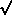R / (1 - R) where R is the reflectance (R < 1). [McL97] FIR Far Infrared FIRAS Far-Infrared Astronomical Spectrometer [LLM96] Fireball see Meteor; see also Primeval Fireball. [H76] FIRST Far-Infrared Space Telescope [LLM96] First Order Transition A phase transition is called first order if it occurs in a manner similar to the way water boils. Bubbles of the new phase (steam) form in the midst of the old phase (water), so that temporarily the two distinct phases (steam and water) coexist. In a second order phase transition, by contrast, one phase evolves into the other as the temperature changes, so the two phases never coexist. [G97] First Point of Aries see Vernal Equinox. [H76] Fission In nuclear physics, the splitting of the atomic nucleus of a heavy element, resulting in the emission of nuclear energy and possibly causing a chain reaction (with similar results) within a mass of the element. [A84] Fission, Nuclear Interaction in which nucleons previously united in an atomic nuclei are disjoined, releasing energy. Fission powers "atomic" bombs. Compare with Fusion. [F88] FITS Flexible Image Transport System A method for saving image data which has become standard in astronomy. [McL97] Five-Minute Oscillations Vertical oscillations of the Solar atmosphere with a well-defined period of 5 minutes. [H76] Fixed Point A scale-invariant limit point of the flow of configurations or coupling constants generated by a coarse-graining operation. [D89] FK Fundamental Katalog [LLM96] Flare, Solar Sudden and short-lived (as short as 300 s) brightening of a region of the Solar chromosphere in the vicinity of a sunspot, caused by the sudden release of large amounts of energy (up to 1032 ergs) in a relatively small volume above the Solar "surface." During an intense Solar flare (electron density 1011 compared with 108 in Solar quiet times) the ionization in Earth's atmosphere may increase by several orders of magnitude. Solar flares are classified on a scale of importance ranging from 3+ (largest area) to 1- (smallest area). The largest Solar flares eject a mass of about 1016 g at a speed of roughly 1500 km s-1. [H76] Flare Star (a) Dim red dwarf star that suddenly lights up with great - but brief - luminosity, corresponding to an equally powerful but short-lived burst of radio emission. The cause is thought to be a sudden and intense outburst of radiation on or above the star's surface. [A84] (b) A star that emits flares, which can outshine the entire star. Many red dwarfs are flare stars. (sometimes called UV Ceti star[C95] (c) A member of a class of dwarf stars (usually dM3e-dM6e) that show sudden, intense outbursts of energy. The flares are usually rare and very short with mean amplitudes of about 0.5-0.6 mag. All known flare stars are intrinsically faint and have emission lines of H I and Ca II. It is generally believed that flares in flare stars have certain properties in common: rapid rise to peak light followed initially by a rapid decline and later by a slower phase that occasionally does not return to a preflare level within practical monitoring times (several hours). An increase in radio emission is often detected simultaneously with the optical outburst. About 30 flare stars are known, all within 20 pc. (In at least one theory, the flare star stage directly follows the T Tauri stage.) [H76] (d) Stars undergoing erratic jumps in brightness (up to a few magnitudes) on time scales of the order of minutes. During the quiescent phase the spectrum is that of an M dwarf with emissions in the CaII and Balmer lines. [JJ95] Flash Gate An ultra-thin transparent electrode across the entire back surface of certain CCDs used to control the charge on the back surface and hence the QE for blue and UV light. [McL97] Flash Spectrum An emission spectrum of the olar chromosphere, obtained by placing an objective prism in front of the telescopic lens the instant before (or after) totality in a olar eclipse. [H76] Flat Subject to the rules of geometry codified by Euclid; a shape, like the surface of a perfectly smooth tabletop, and its higher-dimensional generalizations. [G99] Flat Space A synonym for ordinary Euclidean space. [Silk90] Flat Field Or flat-fielding. An extensive, very uniformly illuminated source of light used to determine the relative sensitivity of an imaging detector composed of many picture elements (pixels) each with a slightly different response to light. The correction process is called flat-fielding. [McL97] Flat Universe (a) A Universe in which there is no curvature to the spacetime continuum. This means that the kinetic energy of the expansion is exactly balanced by the potential gravitational energy of the matter. Thus, after an infinite amount of time the Universe will stop expanding. [C97] (b) A homogeneous, isotropic Universe is called flat if it is just on the borderline between being spatially closed and spatially open, so the geometry is precisely Euclidean. If Einstein's cosmological constant is zero, then a flat Universe will go on expanding forever, but the velocity of recession between any two objects would approach zero at large times. [G97] (c) A Universe that is at the boundary between an open and closed Universe. In a flat Universe, the average mass density always has precisely the critical value. A flat Universe has zero total energy and an infinite size. Flat Universes have the geometry of an infinite, flat surface, that is, Euclidean geometry. The value of omega is 1 for a flat Universe. (see Closed Universe; Critical Mass Density; Euclidean Geometry; Omega; Open Universe.) [LB90] Flatness Problem(a) Poses the question: Why, out of an infinite number of possibilities, is our Universe so close to the one special case: the "flat" Universe? [C97] (b) The riddle of why the Universe is neither dramatically open nor closed, but appears to be almost perfectly balanced between these states. [F88] (c) A problem of the traditional big bang theory (without inflation) related to the precision required for the initial value of omega, the ratio of the actual mass density to the critical mass density. If the description is started at one second after the big bang, for example, omega must have been equal to one to an accuracy of fifteen decimal places, or else the resulting Universe would not resemble our own. Yet the traditional big bang theory offers no explanation for this special value, which must be incorporated as an arbitrary postulate about the initial conditions. (see also Horizon Problem) [G97] (d) The puzzle of why the Universe today is so close to the boundary between open and closed, that is, why it is almost flat. Equivalently, why should the average mass density today be so close to the critical mass density, but not exactly equal to it? If omega begins bigger than 1, it should get bigger and bigger as time goes on; if it begins smaller than 1, it should get smaller and smaller. For omega to be near 0.1 today, about 10 billion years after the big bang, it had to be extraordinarily close to 1 when the Universe was a second old. Some people consider such a fine balance to have been highly unlikely according to the standard big bang model, and thus are puzzled as to why the Universe today is almost flat. (see Closed Universe; Critical Mass Density; Flat Universe; Open Universe.) [LB90] Flattening A parameter that specifies the degree by which a planet's figure differs from that of a sphere; the ratio f = (a - b) / a, where a is the equatorial radius and b is the polar radius. [S92] Flavor (a) The term used to describe different quark types. There are six quark flavors: up, down, strange, charm, bottom and top. [CD99] (b) Whimsical name given to the quality that distinguishes the six known varieties of quarks. These flavors have the names up, down, strange, charmed, top (or truth) and bottom (or beauty). The six different leptons are also sometimes described using the term flavor. [D89] (c) Designation of quark types - up down, strange, charmed, top, and bottom. Flavor determines how the weak nuclear force influences quarks. [F88] (d) The known quarks exist in six different types, or flavors: up, down, charmed, strange, top, and bottom. The up and down quarks belong to the first generation, the charmed and strange quarks belong to the second, and the top and bottom quarks belong to the third. The up, charmed, and top quarks each have an electrical charge 2/3 that of a proton, while the down, strange, and bottom quarks have a charge -1/3 that of a proton. [G97] (e)In particle physics, another word for "type". For example, there are 6 flavors of quarks, meaning that there are 6 different types of quarks. [LB90] Flickering Aperiodic behavior in an oscillating system; rapid, large-amplitude variations in light. [H76] Flocculus see Plage [H76] Flop Transition Evolution of the Calabi-Yau portion of space in which its fabric rips and repairs itself, yet with mild and acceptable physical consequences in the context of string theory. [G99] Fluctuations (a) Spontaneous deviations of the macroscopic variables from a certain `reference' state, arising from the thermal motion and the interactions of the molecules. [D89] (b) Deviations from uniform conditions. For example, a mass of gas that bunches up to a higher density than the surrounding gas would be referred to as a fluctuation. The amount of bunching at each mass scale is called the fluctuation spectrum. Most cosmologists attempt to explain the observed structures in the Universe (such as groups of galaxies) by the gravitational condensation and growth of small fluctuations of mass in the past. [LB90] Fluorescence (a) The absorption of energy by atoms, molecules, etc., followed by immediate emission of electromagnetic radiation as the particles make transitions to lower energy states. Fluorescence is a type of luminescence in which the emission of radiation does not persist after the exciting source has been removed. The excited states have very short lifetimes. Fluorescence, like luminescence, can be produced in a number of ways. Examples are by bombardment with electrons and by absorption of other electromagnetic radiation. In the case of electromagnetic radiation the emitted radiation often has lower frequency than the absorbed radiation. A particular example is the absorption of ultraviolet radiation followed by emission of visible radiation. This effect is used in fluorescent paints and in 'fabric brighteners' added to detergents. The inside coating of fluorescent tubes is another example of a material that converts ultraviolet radiation (from the discharge) into visible radiation. (see also Luminescence) [DC99] (b) The absorption of a photon of one wavelength and reemission of one or more photons at longer wavelengths, especially the transformation of ultraviolet radiation into visible light. [H76] (c) The emission of light at one wavelength, the green say, following absorption of light with a much shorter wavelength such as the ultraviolet. The UV photon parts with its energy by ejecting the electron into a high-energy level from which it cascades back down, releasing photons of lower energy and therefore longer wavelengths. A material which has this property is called a phosphor. [McL97] FluorineA slightly greenish-yellow highly reactive gaseous element belonging to the halogens. It is slightly more abundant than chlorine, accounting for about 0.065% of the Earth's crust. Fluorine is the most reactive and most electronegative element known; in fact it reacts directly with almost all the elements, including the rare gas xenon. Symbol: F; b.p. -188.14°C; m.p. -219.62°C; d. 1.696 kg m-3 (0°C); p.n. 9; r.a.m. 18.99840.32. [DC99] Flux (a) Total radiation going out from the 2solid angles of a hemisphere. If the radiation is uniform, the flux is 2times the intensity. Measured in ergs cm-2 s-1. [H76] (b) In magnetism, the total number of lines of magnetic force passing through a specified loop. [D89] (c) A measure of the amount of power or radiation received per unit time per unit area measured in erg cm-2 s-1. [Silk90] Flux Density Flux of radiation through a unit surface; the strength of an electromagnetic wave, defined as the amount of power incident per unit area. In radio astronomy, the brightness temperature integrated over the solid angle of the source yields the flux density. [H76] Flux Tube A tube of magnetic field lines. [H76] Flux Unit (a) Unit of flux density. 1 f.u. = 10-26 watts per square meter per hertz (see Jansky). [H76] (b) In radio astronomy energy is usually measured in units of 10-26 W m-2 Hz-1. The factor seems to have been first used in 1951; it is interesting to note that radio astronomers have always measured energy flux in terms of square metres. (see Jansky) [JM92] FOC Faint Object Camera (Hubble Space Telescope). [LLM96] Focal Plane Scale The relationship between angles on the sky, in seconds of arc, and millimeters of size at the focus of the telescope; i.e. the number of arcsecs per millimeter. [McL97] Focal Ratio The ratio of the focal length (F) of a mirror or lens to its diameter (D) expressed as a number; f/# = F/D. Also defines the cone angle of the beam. Small focal ratios e.g. f/# = F/D = 1 are said to be "fast" and represent a very large cone angle. Large focal ratios e.g. f/# = 35 are said to be "slow" and indicate a very small cone angle. [McL97] Focal Reducer An optical component or system for changing the image scale of a telescope to achieve a better match between the seeing disk and the pixel size. (see Optical Matching) [McL97] Fokker-Planck Equation A modified form of the Boltzmann equation allowing for collision terms in an approximate way. It is used in the problem of charged-particle transport in fluctuating electromagnetic fields. [H76] Fomalhaut (a) The brightest star in the constellation Piscis Austrinus, Fomalhaut is a white A-type main-sequence star 21 light-years away. [C95] (b) An A3 V star about 7 pc distant. [H76] Forbidden Processes can be naively imagined that might occur, but should not occur according to the predictions of the Standard Model. Whether they occur is then a test of the Standard Model. If they occurred at the same rate as other processes the Standard Model would be wrong; if they occur at much smaller rates, or do not occur at all, they provide a clue as to how to extend the Standard Model. None of the processes forbidden by the Standard Model have been observed. [K2000] Forbidden Energy Gap The unoccupiable interval of electron energy levels which forms in a crystalline substance (that is one having a periodic atomic formation) between the valence band (bound electrons) and the conduction band (free electrons). The forbidden energy gap is very large in insulators, non-existent in metals, and small but finite in a semiconductor. [McL97] Forbidden Lines Spectral lines emitted from metastable states, or those which have a very low probability (10-9-10-10) of occurrence. They appear at particle densities108 cm-3. All forbidden lines have low excitation potentials. Forbidden lines are designated by enclosing them in brackets, e.g., [O II]. [H76] Forbush Decrease A decrease in cosmic-ray intensity with an increase in olar activity (and vice versa). This phenomenon was first noted by Forbush in 1954. [H76] Force (a) Agency responsible for a change in a system. In Newtonian mechanics, gravitational force bends the moon away from the straight trajectory it would otherwise pursue. [F88] (b) All the phenomena we know of in nature can be described by five forces: the gravitational, weak, electrical, magnetic, and strong forces. The electrical and magnetic forces are unified into the electromagnetic force. Although the weak and electromagnetic forces appear different to us, they can be described as unified into one force (electroweak) in a more basic way ; there is evidence that a similar unification of the electroweak force with the strong force also occurs. The attempt to unify all forces is an active research area. In particle physics, force and interaction mean essentially the same thing. [K2000] Force Carriers Particles that act as the transmitters of forces. The best known example is the photon, which transmits electromagnetic forces. The gluons are the transmitters of the strong interactions, and the W+, W-, and Z0 particles are the transmitters of the weak interactions. [G97] Force Charge A property of a particle that determines how it responds to a particular force. For instance, the electric charge of a particle determines how it responds to the electromagnetic force. [G99] Formaldehyde H2CO -- An organic molecule, the first polyatomic molecule to be discovered in interstellar space (in 1969). In 1973 It was discovered in two external galaxies. [H76] Formamide HCONH2 -- A molecule discovered in interstellar space in 1971 at 6.5 cm. [H76] Formic Acid H2C02 -- A simple organic acid, the first to be detected in interstellar space (in 1970 at 18.3 cm). Formic acid is the "sting" of an insect. [H76] Fornax 1. A faint constellation in the southern sky. 2. A dwarf galaxy that orbits the Milky Way and lies 440,000 light-years from the Galactic center. It was discovered in 1938. [C95] Fornax A A 10th-magnitude S0 galaxy (NG6 1316), which is a strong radio source. [H76] Fornax System A dwarf spheroidal galaxy, about 190 kpc distant, in the Local Group (Mv-12, M2 × 107 M). [H76] 47 Tucanae A globular cluster in the southern constellation Tucana and a member of the thick-disk population. [C95] Forward (or Reverse) Bias A term applied to an electronic device known as a diode - usually formed by a junction of p-type and n-type semiconductor material - in which current flows easily if the externally applied voltage has the correct polarity or direction. If the opposite polarity is applied there is almost no current flow and the device is said to be reverse biased. [McL97] Fossils Geological remains of what was once a living thing. [F88] Fossil Strömgren Sphere A relict HII region which remains after the evolution of its exciting star. [H76] 4-kpc Arm A component of the Sagittarius arm with non-circular gas motions. (see 3-kpc Arm) [H76] 4N Nuclei Nuclei possessing equal and even numbers of neutrons and protons. 4N nuclei are formed in supernova envelopes at temperatures of at least 2 × 109 K and are very stable. (or Even-Even Nuclei) [H76] Four-Vector A quantity that has four components which, under the Lorentz transformation, transform like space and time. Instead of locating a point in three-dimensional space, a spacetime four-vector locates a point in four-dimensional spacetime. (four-dimensional vector) [H76] Fourier Analysis The analysis of a periodic function into its simple harmonic components. [H76] Fourier Component A measure of the fluctuations of some physical quantity on a particular length scale. The density of sand on a beach, for example, would have a small Fourier component on the scale of a few feet, where the beach appears smooth, but a large Fourier component on the scale of a hundredth of an inch, where the beach appears grainy owing to individual sand particles. [LB90] Fourier Theorem Any finite periodic motion may be analyzed into components, each of which is a simple harmonic motion of definite and determinable amplitude and phase. [H76] FOV Field of View Four-Wave Mixing this is the combination of three optical waves to generate a fourth. It occurs in many optical media provided frequencies and intensities are carefully chosen. It is widely used in phase-conjugation. [D89] FP Fabry-Perot [LLM96] Frame of Reference A set of axes to which positions and motions in a system can be referred. [H76] Francium A radioactive element of the alkali-metal group. It is found on Earth only as a short-lived product of radioactive decay, occurring in uranium ores in minute quantities. A large number radioisotopes of francium are known. Symbol: Fr; m.p. 27°C; b.p. 677°C; p.n. 87; most stable isotope 223Fr (half-life 21.8 minutes). [DC99] Franck-Condon Principle A theoretical interpretation of the relative intensity of vibrational transitions in an electronic band on the assumption that the intense transitions correspond to situations where an endpoint in the lower vibrational level is vertically below the corresponding endpoint in the upper vibrational level. [H76] Fractal (a) A geometric figure in which a pattern is repeated ad infinitum on smaller and smaller scales. A classic example is Von Koch's snowflake, for which the construction begins with an equilateral triangle. Trisect each side, and replace the middle section by two sides of a smaller equilateral triangle, bulging outward. The snowflake is obtained by repeating this process for each side of the resulting figure, then for each side of the subsequent figure, and continuing forever. [G97] (b) A pattern that repeats itself or nearly repeats itself on many different scales of magnification. For example, suppose that some ink on a piece of paper appears to form a star. If you look at the piece of paper with a magnifying glass, you see that the dark areas are not solid black, but are formed of tiny stars themselves. If you look at one of these small stars with a microscope, you see that the dark areas of each of the tiny stars is formed from an arrangement of even tinier stars. Such a repeating pattern of stars would be called a fractal. [LB90] Fractal Geometry generalisation of Euclidean geometry suitable for describing irregular and fragmented patterns. A noninteger `fractal dimension' can frequently (but not always) be associated with such patterns. [D89] Frame Transfer A CCD construction in which one half of the imaging area of the device is purposely covered with a mask opaque to light to provide a temporary charge storage section. [McL97] Fraunhofer Diffraction Diffraction observed with incident parallel light. In Fraunhofer diffraction the wavefronts are parallel. Although a special case of Fresnel diffraction, it is far more important in most practical cases. Thus it is used to explain single - and multiple - slit patterns, as well as those produced by circular holes. [DC99] Fraunhofer Lines (a) The dark lines in the spectrum of light from the Sun, caused by the absorption of particular wavelengths by certain elements in its cooler outer regions. The wavelengths of these lines are used as reference points in specifying quantities that vary with wavelength, e.g. refractive constants. [DC99] (b) Absorption lines in the spectrum of the Sun, studied by Fraunhofer in 1814. The nine most prominent he labeled with capital letters (from the red end) A. B. C. D. E. F. G. H. and K. The A and B bands (at 7600 and 7100 Å) are now known to be groups of telluric lines due to O2 absorption in Earth's atmosphere, and C and F are respectively known as Hand H. [H76] Free (of a particle) Not bound to a nucleus. [H76] Free Spectral Range A term used in spectrometers to indicate the wavelength interval between occurrences of the same wavelength produced in the next order of interference or diffraction. [McL97] Free-Fall A collapse in which gas clouds do not hit or impede one another. According to ELS (Eggen, Lynden-Bell & Sandage), the Galactic halo formed in a free-fall collapse. [C95] Free-Free Radiation The acceleration of an unbound (or free) electron by a proton or atomic nucleus results in the emission of electromagnetic radiation. [Silk90] Free Parameter A number which is needed to define a theory well enough so that predictions can be made, but which must be determined by experiment or observation. [G97] Frequency (a) The frequency of a periodic or harmonic motion which repeats itself in equal time units is the number of oscillations or cycles per unit of time. Its unit is the hertz (Hz). [D89] (b) The number of peaks (often called crests) of a propagating wave that cross a given point in a unit of time. For example, if 1000 peaks cross a given point in one second, one says that the frequency is 1000 cycles per second or 1000 hertz. [G97] (c) The number of complete wave cycles a wave completes each second. [G99] (d) The number of oscillations or wave cycles per second passing a given point. For electromagnetic radiation, the product of the frequency and the wavelength is the speed of light. [McL97] Frequency Distribution A statistical arrangement of numerical data according to size or magnitude (see also Distribution Function). [H76] Frequency Standard A generator whose output is used as a precise frequency reference; a primary frequency standard is one whose frequency corresponds to the adopted definition of the second (see Second; Système International), with its specified accuracy achieved without calibration of the device. [S92] Fresnel Diffraction Diffraction observed when either source or screen (or both) are close to the diffractor. In Fresnel diffraction, the wavefronts are not plane (as in Fraunhofer diffraction) and analysis is difficult. The approach is useful in explaining (for instance) diffraction around a circular obstacle. [DC99] Fresnel Lens A type of lens with one surface cut in steps so that transmitted light is refracted just as if by a much thicker (and heavier and more expensive) conventional lens. Lighthouse lamp lenses have long been of this type. Very cheap plastic Fresnel lenses now have many uses, for example in overhead projectors, flashlamps, etc. The angle of each step is made to produce the desired effect. [DC99] Fried Parameter A measure of the scale of the turbulence in the atmosphere. The length over which a disturbance to a wavefront is well-correlated. [McL97] Friedmann Equation (a) An equation for the evolution of the Universe. The Friedmann equation can be derived from Einstein's theory of gravity, plus the assumptions that the Universe is homogeneous (looks the same at every point) and isotropic (looks the same in every direction). The solution of the Friedmann equation tells, among other things, how the distance between galaxies changes with time. (see Homogeneity; Isotropy) [LB90] (b) An equation that expresses energy conservation in an expanding Universe. It is formally derived from Einsteins field equations of general relativity by requiring the Universe to be everywhere homogeneous and isotropic. [Silk90] Friedmann-Lemaître Universes The three standard Big Bang models that were formulated by Friedmann and Lemaître. [Silk90] Friedmann Models A general class of cosmological models that assume the Universe is homogeneous and isotropic on large scales and that allow the Universe to evolve in time. Most calculation in the standard Big Bang model assume a Friedmann cosmology. (see Friedmann Equation; Homogeneity; Isotropy.) A cosmological model that has the same properties as a Friedmann model under some conditions is said to have a Friedmann limit. [LB90] Friedmann Universe A homogeneous, isotropic model of the Universe involving nonstatic (i.e., expanding or contracting) solutions to Einstein's field equations (with zero cosmological constant) calculated by the Russian mathematician A. Friedmann in 1922. [H76] Fringes (a) The light and dark bands obtained by interference or diffraction of light. [DC99] (b) Successive dark and light lines, caused by light beams that are out of phase alternating with those that are in phase. Interference fringes can be used to calculate the apparent diameter of a star. [H76] Fringing The appearance of complex light and dark contours in a CCD image due to constructive and destructive interference effects of light reflected inside the detector. [McL97] Froissart Bound If in a hadron-hadron collision the absorption is complete, then the interaction radius cannot increase faster than the logarithm of the energy. [H76] Froude Number (Fr) A non-dimensional parameter, sometimes called a Froude constant, used in fluid dynamics to describe the flow of a fluid in which there is a free surface. It is defined by the relationship Fr = v/(gl)1/2, where Fr is the Froude number, v is the velocity of the liquid, g the acceleration due to gravity and l is a parameter representing length. The flow of an inviscid, incompressible fluid in two geometrically similar flow systems is dynamically similar when their Froude numbers are the same. The number is named after William Froude (1810-1879), who derived it in 1869. It is used extensively by naval architects. In France it is called the Reece number after F. Reece (1805-1884), a French naval officer from Alsace. All Froude's original notebooks, together with those of his son R. E. Froude (1846-1924), are in the Admiralty Establishment in Gosport, Hampshire. The number 1/Fr2, which identifies the stability of flow in terms of velocity gradient at a fixed surface, is sometimes called the Richardson number (Ri) after Colonel A. R. Richardson of London University, who was very prominent in fluid mechanical research immediately before and after the 1914-1918 war. Low values of Ri indicate the fluid is homogeneous. [JM92] Frozen-In An adjective which applies to the abundance of elements produced by the r-process in a supernova when the temperature has dropped below the point at which they can serve as seed nuclei for further nucleosynthesis. It also applies to the magnetic field lines of a star in gravitational collapse. [H76] F-Type Having a spectral type of F, that is, yellow-white, like Canopus and Procyon. [C95] FT Fourier Transform [LLM96] FTP File Transfer Protocol FTS Fourier Transform Spectrometer [LLM96] FU Flux Unit [LLM96] FU Ori Stars or Fuors A subgroup of T Tau stars with considerable changes in brightness. The post-eruption spectrum is that of a late supergiant. [JJ95] Full Width at Half-Maximum FWHM -- The full width of a spectral line at half-maximum intensity. [H76] Fundamental Constants Physical quantities, like the speed of light or the mass of an electron, that enter into the laws of physics in a basic way and are believed to be the same at all times and everywhere in the Universe. Most physicists take the fundamental constants of nature as given properties of the Universe. [LB90] Fundamental Frequency The lowest characteristic frequency of oscillation of a dynamical system. [H76] Fundamental InteractionsFundamental Stars Stars for which coordinates have been determined to a very high degree of accuracy. [H76] Funneling The concentration of stars from different parts of the main sequence in the red-giant region. [H76] Fusion (a) In nuclear physics, the combining of the atomic nuclei of lighter elements to form nuclei of a heavier element. Such a process involving the atomic nuclei of elements lighter than iron is accompanied by the emission of energy; for fusion of heavier elements, energy must be supplied. The process is thought to contribute to the condensation of stars from interstellar gas and dust. (see also Nuclear fusion) [A84] (b) Interaction in which nucleons are forged together, creating new atomic nuclei and releasing energy. Fusion powers "hydrogen" bombs. [F88] Future Light Cone see Light Cone [H76] FWHM Full Width at Half Maximum The full width of a profile (e.g. the seeing profile or a filter transmission curve) between the two points where the value is 50% of the peak value. [McL97]